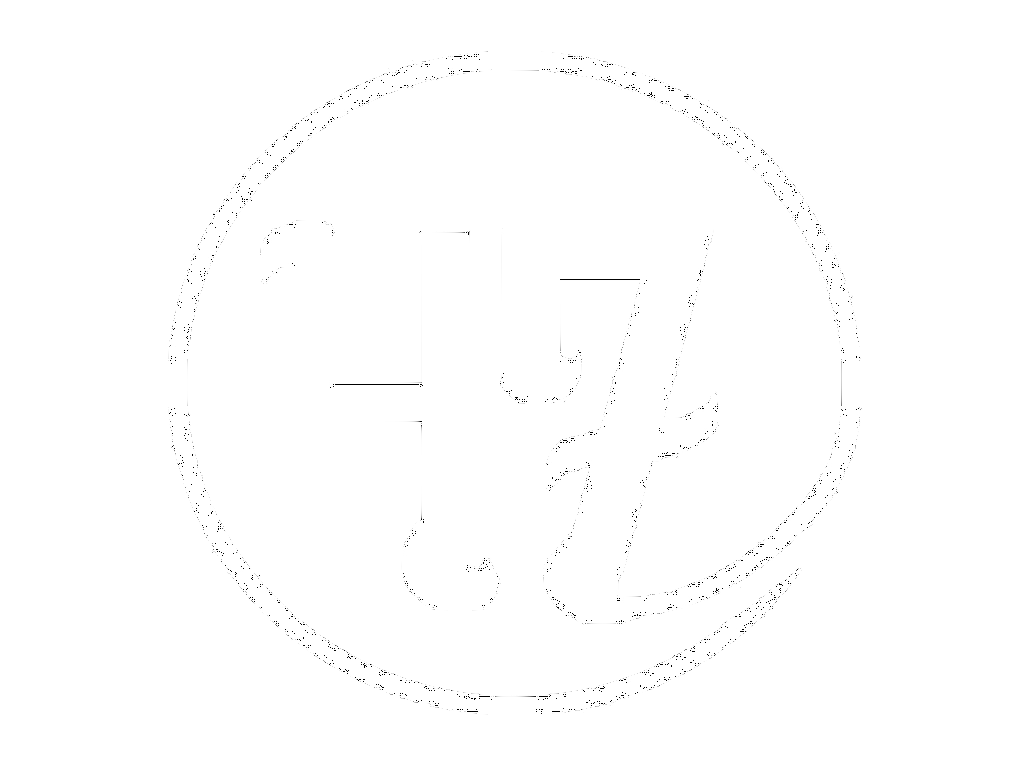Abstract
Libfreenect
Results

# DORi's functionality is messy and complicated, but here is a brief look at how it sees a victim in the pool. To get the full picture of how it works, You can check out DORi in it's entirety here: JackalopeCapstone

The bulk of what we use is from a library called pylibfreenect2, which provides a way to communicate with a kinect V2 using python. Microsoft provides a skeleton tracking SDK for PC based systems, but using a linux system left us to our own devices. This function grabs a very basic skeleton by finding the right most and left most points that are within a calculated body of points. The depthArray passed in is all the points in the frame that are within a certain range of the calculated mean depths for each frame. It returns the data representation of a basic 't' shape, which represents the spine and the width of the target data
```    def getSkeleton(self, depthArray, average, rows, cols):
topY = 0
leftX = rows
bottomY = cols
rightX = 0

for d in depthArray:
if (d['x'] > rightX):
rightX = d['x']
if (d['x'] < leftX):
leftX = d['x']
if (d['y'] < bottomY):
bottomY = d['y']
if (d['y'] > topY):
topY = d['y']

averageX = (leftX + rightX) / 2
returnValues = {'averageX': averageX,
'topY': topY,
'bottomY': bottomY,
'rightX': rightX,
'leftX': leftX}

return returnValues
```
The point ravel function is used for depth frames from the kinect, and essentially turns a 1 dimensional array into an array of 3 dimensional points. The frame is stored in 1 dimension, so to get the depth with the associated x and y coordinates, an iterative loop goes through the rows and columns and multiplies the sum by the total rows. Shiffman.net explains this in depth here , but essentially the multiplication by rows sets how far into the array the point basically resides, and the addition of the col and row set it's exact location. Again, this is a very rudimentary explanation, but enough to follow the code. Returned is the depth at a point (x,y), plus the x and y coordinates. This makes using the depth array data much easier later on.
```    def pointRavel(self, unraveledArray, rows, cols):
raveledArray = []
d = unraveledArray.ravel()
for row in range(rows):
for col in range(cols):
try:
offset = row + col * (rows)
raveledArray.append({'x': row, 'y': col, 'depth': d[offset]})
except IndexError as e:
print e

return raveledArray
```
This data loop occurs for each frame the kinect returns. The registration has to happen to grab a new frame, and is used to get the depth array that is analyzed. The depthArray is an object from the pylibfreenect2 library, and has the attribute shape, which returns rows and columns. Then, the pointRavel returns our new more readable depth array, which is used to calculate a mean depth. the mean depth is used get a basic body shape, which is just an array of points which have a depth within a range of the average depth. In a pool, this gives us a really good approximation of a person's full body. Using this body array, we run the above skeleton function to find the spine. This will be used in the checkDrowning function.
```    def fullDataLoop(self):

registration = Registration(self.device.getIrCameraParams(),
self.device.getColorCameraParams())

depthArray = self.update(registration)
rows, cols = depthArray.shape
d = self.pointRavel(depthArray, rows, cols)
m = self.getMeanDepth(d, rows, cols)
b = self.getBody(d, m, rows, cols)
s = self.getSkeleton(deptharraytest, m, cols, rows)

return {'spine' : s, 'meanDepth' : m}
```
This function is the main loop of the py file, and is what is called in the rest of our processes. it takes a set time limit (set as 20 seconds in the settings), and for that duration runs the data loop we explored above. The spine is checked for a minimum threshold of movement, if it's discovered that the spine spine hasn't made meaningful movement in 20 seconds, DORi is alerted to a potential drowning. If not, the device simply stops and waits to be started again
```    def checkDrowning(self):
print "checkDrowning"
drowningRisk = True
drowning = False
falsePositive = 0
# 20 seconds from start of def #
timeLimit = time.time() + DROWN_TIME
self.device.start()
while drowningRisk:
print 'checking...'
if time.time() > timeLimit:
drowningRisk = False
drowning = True

data = self.fullDataLoop()
if (self.valueUnbounded((self.averageSpineX - data['spine']['averageX']),
X_CHANGE_THRESHOLD)):
falsePositive += 1
if falsePositive > 100:
drowningRisk = False
else:
continue
if drowning:
self.device.stop()
print "This guy is for sure drowning"
sys.exit(47)

self.device.stop()
```
Kinect's depth cloud viewDORi in action